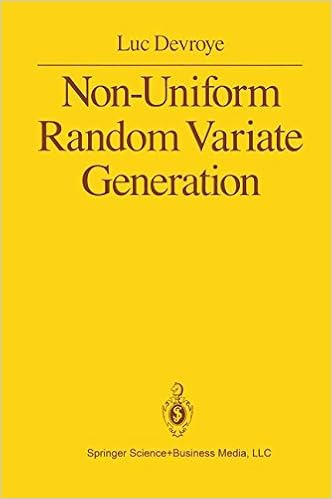## ratio-of-uniformsOne approach to random number generation that had always intrigued me is Kinderman and Monahan’s (1977) ratio-of-uniform method. The method is based on the result that the uniform distribution on the set A of (u,v)’s in R⁺xX such that

0≤u²≤ƒ(v/u)

induces the distribution with density proportional to ƒ on V/U. Hence the name. The proof is straightforward and the result can be seen as a consequence of the fundamental lemma of simulation, namely that simulating from the uniform distribution on the set B of (w,x)’s in R⁺xX such that

0≤w≤ƒ(x)

induces the marginal distribution with density proportional to ƒ on X. There is no mathematical issue with this result, but I have difficulties with picturing the construction of efficient random number generators based on this principle.I thus took the opportunity of the second season of [the Warwick reading group on] Non-uniform random variate generation to look anew at this approach. (Note that the book is freely available on Luc Devroye’s website.) The first thing I considered is the shape of the set A. Which has nothing intuitive about it! Luc then mentions (p.195) that the boundary of A is given by

u(x)=√ƒ(x),v(x)=x√ƒ(x)

which then leads to bounding both ƒ and x→x²ƒ(x) to create a box around A and an accept-reject strategy, but I have trouble with this result without making further assumptions about ƒ… Using a two component normal mixture as a benchmark, I found bounds on u(.) and v(.) and simulated a large number of points within the box to end up with the above graph that indeed the accepted (u,v)’s were within this boundary. And the same holds with a more ambitious mixture:### One Response to “ratio-of-uniforms”

This site uses Akismet to reduce spam. Learn how your comment data is processed.